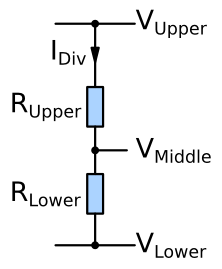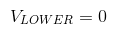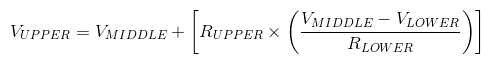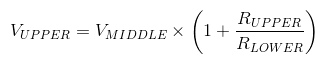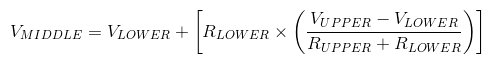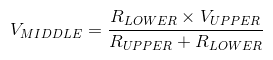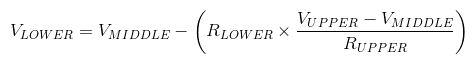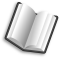# The Lab Book Pages

An online collection of electronics information

Dr. Andrew Greensted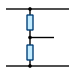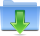PotentialDivider.jar

# Potential Divider

The Potential Divider Search Tool can be used for finding a pair of resistor values that give the correct voltages in a potential divider circuit. It will also calculate the voltages in a potential divider circuit given the resistor values. The application can be started using Java Web Start by clicking the Launch button below. Alternatively, a executable Jar file can be downloaded using the link above.

## Application Example

The image below is a typical voltage regulator circuit. It shows the resistor feedback network used to set the output voltage. The problem is to find a pair of resistor values that will give the correct output voltage VOUT for the regulator's feedback voltage VFB.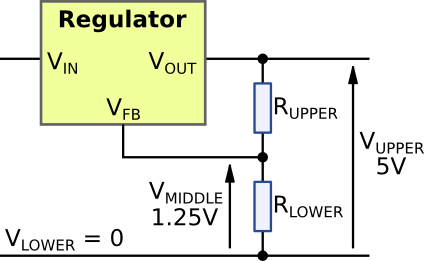The search tool outputs a table like the one shown below. In this case the resistor values were constrained to the region 10kΩ to 1MΩ with a 1% tolerance. The software will output up to 20 results ordered by their accuracy of meeting the required voltages. The table below just shows the top 4.

The table also shows the minimum and maximum output voltage and resistor values due to resistor tolerances.

VUPPER (V) VMIDDLE (V) VLOWER (V) RUPPER (Ω) RLOWER (Ω) IDIV (A) PRUPPER (W) PRLOWER (W)
5.0
(4.93 to 5.08)
1.25
0
33.00k
(32.67k to 33.33k)
11.00k
(10.89k to 11.11k)
113.64μ
426.14μ
142.05μ
5.0
(4.93 to 5.08)
1.25
0
330.00k
(326.70k to 333.30k)
110.00k
(108.90k to 111.10k)
11.36μ
42.61μ
14.20μ
5.0
(4.93 to 5.08)
1.25
0
39.00k
(38.61k to 39.39k)
13.00k
(12.87k to 13.13k)
96.15μ
360.58μ
120.19μ
5.0
(4.93 to 5.08)
1.25
0
390.00k
(386.10k to 393.90k)
130.00k
(128.70k to 131.30k)
9.62μ
36.06μ
12.02μ

The table also shows the current that will flow through the network for a given pair of resistor values. This is useful information for deciding which result to use. The calculator assumes no current is flowing into the VFB pin, therefore to get an accurate result, pick a result were the network current is at least 100 times the feedback pin current.

The power columns show the power that will be dissipated in each resistor. This is useful for deciding each resistor's package size.

## Divider Equations

The equations below can be used for calculating the voltage values in a potential divider given the resistor values.